## In a hydraulic lift, if you exert a downward force of 100 N on a piston with an area of 1 m^3, how much upward force would be exerted on a p

Question

In a hydraulic lift, if you exert a downward force of 100 N on a piston with an area of 1 m^3, how much upward force would be exerted on a piston with an area of 20 m^3?

in progress 0
2 months 2021-07-23T17:29:43+00:00 1 Answers 0 views 0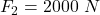Explanation:

We have,

Area 1,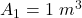Force 1,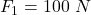Area 2,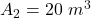It is required to find the force 2. In a hydraulic lift, the pressure at two points is same. It means :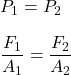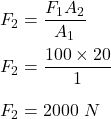So, second force is 2000 N.